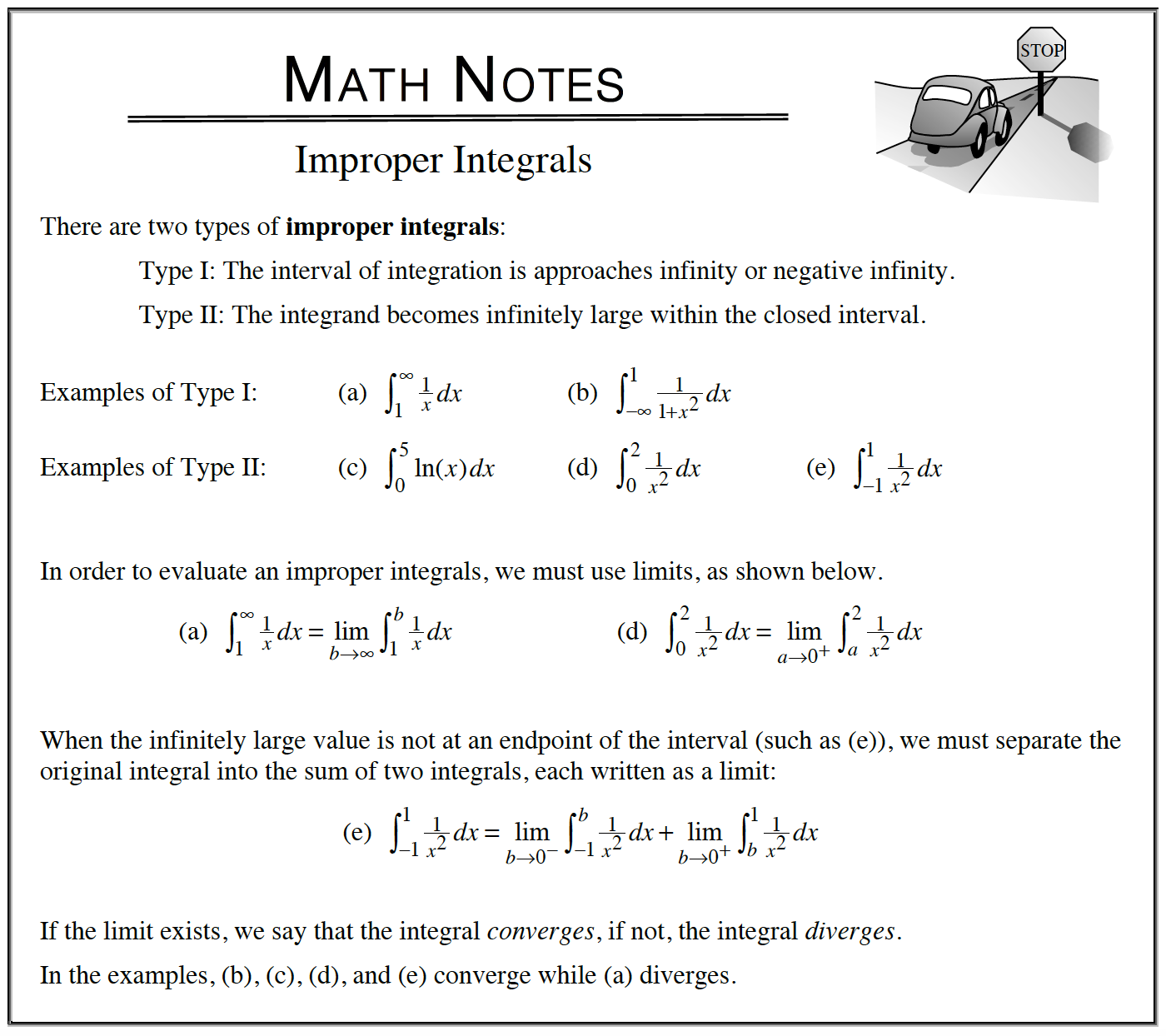### Home > APCALC > Chapter 6 > Lesson 6.5.1 > Problem6-167

6-167.

Determine what makes each of the integrals below “improper.” Use a limit to rewrite the integral, then evaluate. If the integral diverges, say so. Homework Help ✎1. $\int _ { 0 } ^ { \infty } e ^ { - x } d x$

Does the graph of the integrand, $y = e^{-x}$, have any asymptotes on the bounded domain, $[0, ∞)$?

$\lim_{a\to \infty }\int_{0}^{a}e^{-x}dx=\lim_{a\to \infty }-e^{-x}\Big|_0^a=\underline{\ \ \ \ \ \ \ }$

The answer should be very pleasing.

2. $\int _ { - 3 } ^ { 2 } \frac { 1 } { 2 x + 6 } d x$

Does the graph of the integrand, $y= \frac{1}{2x-6}$ have any asymptotes on the bounded domain, $[−3, 2]$?

3. $\int _ { 1 } ^ { \infty } \frac { \operatorname { ln } ( x ) } { x } d x$

Before you rewrite as a limit, use U-substitution on the integrand and the bounds. Let $U = \ln(x)$.